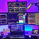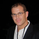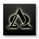# CM ATR Stops/Bands - Multi-TimeFrame

6547 obejrzeń
CM_MTF ATR Bands/Stops

Many Options Available Via Input Tab:
-Chart Defaults to Upper and Lower ATR's Based on Current Chart TimeFrame
-Ability to Plot either Upper and/or Lower ATR's
-Ability to Change the Time Frame ATR's are Based On!
-Ability to change Look Back Period and ATR Multiplier Individually for Both Time Frames
-This Gives you the ability to plot same Time Frame with (for ex.) a 5 ATR with a 1.5 Mult and a 14 ATR with a 2.0 Mult etc.
-Or you can plot Daily ATR's on a 60 minute Chart etc.
-ATR Multipliers are Calculated with Code that allows "Non Whole Numbers" Allowing Ability to use 1.5 ATR's, 1.8 ATR's etc.

***Endless # of Combinations can be used!!!!
```//Created By ChrisMoody on 11-20-2014

study(title="CM_MTF_AverageTrueRange_Stops", shorttitle="CM_MTF_ATR_Stops", overlay=true)
length = input(5, minval=1, title="# of Bars to Calculate ATR")
mult = input(15, minval=1, maxval=40, title="Value X .1, 10=1 (No Multiplier), 15=1.5 times ATR Value")
sav = input(true, title="Show ATR Stop Above Price")
sbv = input(true, title="Show ATR Stop Below Price")
useCurrentRes = input(true, title="Use Current Chart Resolution?")
resCustom = input(title="Use Different Timeframe? Uncheck Box Above", type=resolution, defval="D")
//Optional 2nd ATR Bands Inputs
def = input(false, title="Optional 2nd ATR Bands - Inputs Below?")
length2 = input(5, minval=1, title="# of Bars to Calculate ATR - Optional 2nd Bands Plot")
mult2 = input(30, minval=1, maxval=40, title="Value X .1, 10=1 (No Multiplier), 15=1.5 times ATR Value - Optional 2nd ATR Bands Plot")
sav2 = input(false, title="Show ATR Stop Above Price - Optional 2nd ATR Bands Plot")
sbv2 = input(false, title="Show ATR Stop Below Price - Optional 2nd ATR Bands Plot")
useCurrentRes2 = input(true, title="Use Current Chart Resolution - Optional 2nd ATR Bands Plot?")
resCustom2 = input(title="Use Different Timeframe - Optional 2nd Bands Plot? Uncheck Box Above", type=resolution, defval="D")

res = useCurrentRes ? period : resCustom
res2 = useCurrentRes2 ? period : resCustom2

//Multiplier Calculation
Xmult = mult * .1
//Optional 2nd Bands
Xmult2 = mult2 * .1

//ATR Calculation with Multiplier
atrMult = sma(tr, length) * Xmult
outATR = security(tickerid, res, atrMult)
//Optional 2nd Bands
atrMult2 = sma(tr, length2) * Xmult2
outATR2 = security(tickerid, res2, atrMult2)

//Values Above and Below Bars
aboveStop = high + outATR
belowStop = low - outATR
//Optional 2nd Bands
aboveStop2 = high + outATR2
belowStop2 = low - outATR2

///Values Plotting chosen Chart Resolution
aboveFinal = security(tickerid, res, aboveStop)
belowFinal = security(tickerid, res, belowStop)
//Optional 2nd Bands
aboveFinal2 = security(tickerid, res2, aboveStop2)
belowFinal2 = security(tickerid, res2, belowStop2)

//plot Statements
p1=plot(sav and aboveFinal ? aboveFinal : na, title="1st ATR Band Upper Plot1", style=linebr, linewidth=3, color=red)
p2=plot(sbv and belowFinal ? belowFinal : na, title="1st ATR Band Lower Plot1", style=linebr, linewidth=3, color=lime)
plot(sav and aboveFinal ? aboveFinal : na, title="1st ATR Band Upper Plot2", style=circles, linewidth=3, color=red)
plot(sbv and belowFinal ? belowFinal : na, title="1st ATR Band Lower Plot2", style=circles, linewidth=3, color=lime)
//Optional 2nd Bands
p3=plot(sav2 and aboveFinal2 ? aboveFinal2 : na, title="2nd ATR Band Upper Plot1", style=linebr, linewidth=4, color=red)
p4=plot(sbv2 and belowFinal2 ? belowFinal2 : na, title="2nd ATR Band Lower Plot1", style=linebr, linewidth=4, color=lime)
plot(sav2 and aboveFinal2 ? aboveFinal2 : na, title="2nd ATR Band Upper Plot2",  style=circles, linewidth=4, color=red)
plot(sbv2 and belowFinal2 ? belowFinal2 : na, title="2nd ATR Band Lower Plot2", style=circles, linewidth=4, color=lime)
fill(p1, p3, color=red, transp=80)
fill(p2, p4, color=lime, transp=80)```

## KomentarzeChris. What ever you make when you make is unique, different and super performer. Chris i have a request can you make a supertrend indicator which shows a higher time frame trend and in lower time frame we can take a trade. that will be great help to retailers although there are certain but confusing.
Higher time frame:- Daily, Weekly, Monthly,
Snipper Trading Frame Entry/ Exit:- 15,30, hourly.

Will wait for a positive response.
OdpowiedzNHARISH
I hope you understand...I've known John and Hubert from TTM for a long time. I never take there codes and post them. There are a lot of talented coders in the pine script editor chat that would be willing to help you if you ask them nicely.
OdpowiedzYou are on a roll! Two questions: 1) Could there an option to flip the ATR band with the trend? 2) Could there be more decimal places to the ATR multiple (e.g. 1.618 versus 1.6 - I think this is entered as 16 currently and it translates to 1.6).
OdpowiedzAlphaoptions
The second part is simple...take this part of the code...right under the inputs
Step 1
//Multiplier Calculation
Xmult = mult * .1
//Optional 2nd Bands
Xmult2 = mult2 * .1

The * .1 takes 15 and makes it 1.5 therefore if you changed it to .001 then the multiplier would need to be 1618

So end result would be
//Multiplier Calculation
Xmult = mult * ..001
//Optional 2nd Bands
Xmult2 = mult2 * .001

Step 2
In inputs section take the mult and mult2 Input and change the maxval setting from 40 to as high as you would want it, or you can take that parameter out all together. also set the default input to 1000 or so...or 1618 if thats the default setting your going to use.

End Result: Replace the first two lines in Inputs tab, and then the code a few lines below the inputs tab to this.

mult = input(1618, minval=1000, maxval=5000, title="Value X .1, 1000=1 (No Multiplier), 1618=1.618 times ATR Value")
mult2 = input(1618, minval=1000, maxval=5000, title="Value X .1, 1000=1 (No Multiplier), 1618=1.618 times ATR Value - Optional 2nd ATR Bands Plot")

//Multiplier Calculation
Xmult = mult * ..001
//Optional 2nd Bands
Xmult2 = mult2 * .001

Let me know if it doesn't work...but it should.
OdpowiedzChrisMoody
First band is 1.618, 2nd band is 2.618 using the code above

OdpowiedzChrisMoody
I must have made a mistake. I could not get it to work.
OdpowiedzChrisMoody
Unfortunately it did not like it. It just left me without a plot of anything. I am very new to the group and coding so I may have made a mess of it. I am thinking of using it with the HiLo you posted earlier. It might get a busy chart but it would give a view of trend and the stop / entry, re-entry points. It would be way cool if the two scripts could be merged so that the ATR and its stop plot only when in same direction as HiLo as it would be cleaner (i.e. just on one side). Having these giving the stop, trend, pullback areas along the way to the next target (like your Pivot bands, then it would be quite a complete approach). Pulling all of them together. If used with the bands (as a separate script), it would be even more important to plot the ATR on one side - or so it would seem?
OdpowiedzAlphaoptions
Here is a link to the code...either create a new indicator...or paste it over the current indicator and save it.

http://pastebin.com/ATzGn89L
Odpowiedz